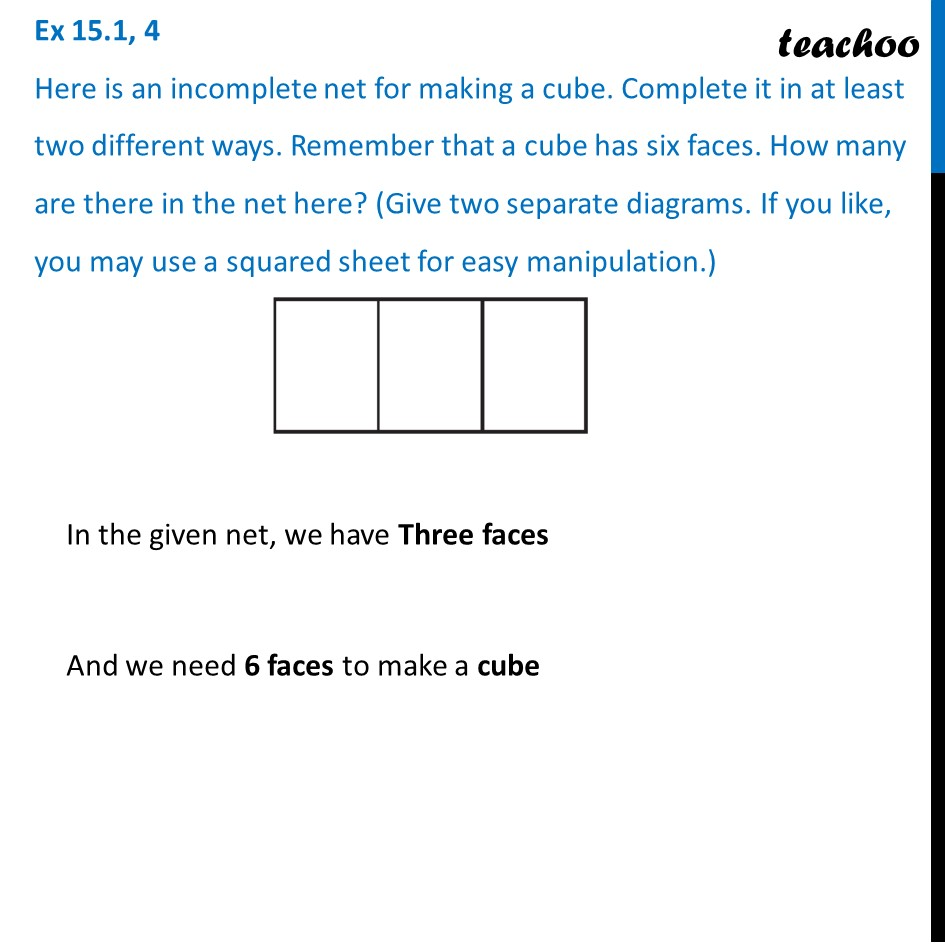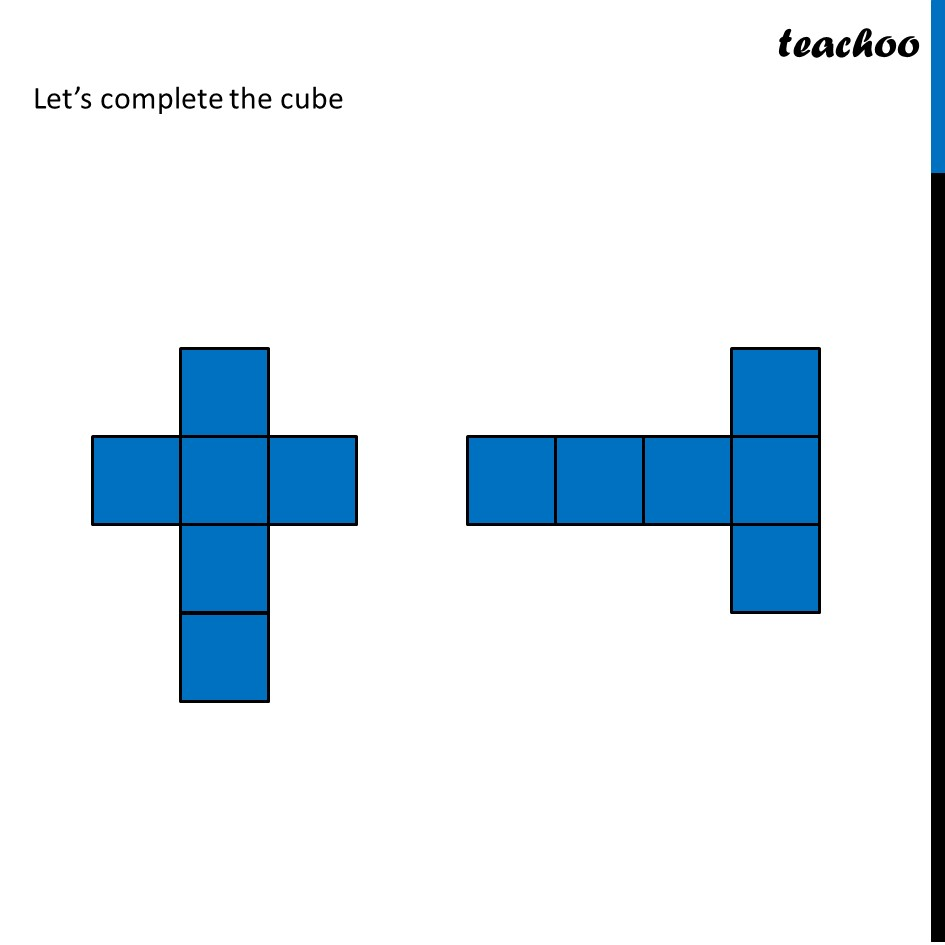Ex 15.1

Chapter 15 Class 7 Visualising Solid Shapes
Serial order wise### Transcript

Ex 15.1, 4 Here is an incomplete net for making a cube. Complete it in at least two different ways. Remember that a cube has six faces. How many are there in the net here? (Give two separate diagrams. If you like, you may use a squared sheet for easy manipulation.) In the given net, we have Three faces And we need 6 faces to make a cube Let’s complete the cube